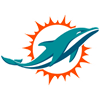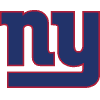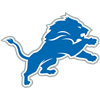DraftKings Make Playoffs Odds
-650
0.15 to 1Buccaneers
82.7% implied probability

-550
0.18 to 1Bills
80.9% implied probability

-500
0.2 to 1Packers
78.9% implied probability

-250
0.4 to 1Rams
68.2% implied probability

-250
0.4 to 1Cowboys
67.8% implied probability

-225
0.44 to 149ers
65.6% implied probability

-215
0.47 to 1Chiefs
64.8% implied probability

-190
0.53 to 1Eagles
62.1% implied probability

-175
0.57 to 1Colts
60.4% implied probability

-160
0.63 to 1Chargers
58.6% implied probability

-150
0.67 to 1Ravens
57.4% implied probability

-150
0.67 to 1Broncos
57.4% implied probability

-135
0.74 to 1Bengals
55.3% implied probability

-110
0.91 to 1Titans
50.0% implied probability

-105
0.95 to 1Vikings
48.9% implied probability

+125
1.25 to 1Saints
42.2% implied probability

+125
1.25 to 1Cardinals
42.6% implied probability

+140
1.4 to 1Dolphins
39.6% implied probability

+150
1.5 to 1Commanders
38.1% implied probability

+160
1.6 to 1Raiders
36.6% implied probability

+160
1.6 to 1Patriots
36.6% implied probability

+225
2.25 to 1Giants
29.5% implied probability

+330
3.3 to 1Steelers
22.3% implied probability

+400
4 to 1Lions
19.1% implied probability

+400
4 to 1Bears
19.1% implied probability

+450
4.5 to 1Jaguars
17.3% implied probability

+500
5 to 1Seahawks
16.1% implied probability

+500
5 to 1Panthers
16.1% implied probability

+700
7 to 1Jets
12.0% implied probability

+800
8 to 1Falcons
10.7% implied probability

+1600
16 to 1Texans
5.7% implied probability